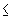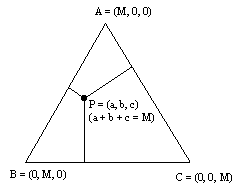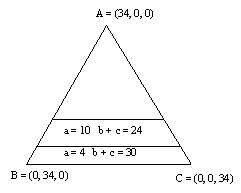## Project 9: The Geometry of the Core of a 3-Person Game

It often happens that when individuals (or towns or companies) cooperate they can achieve savings over what costs would be if they were to proceed on their own. Imagine that there are three individuals A, B, and C and that if they do a certain project on their own the cost to A, B, and C, respectively, would be:

C({A}) = 10; C({B}) = 16; C({C}) = 24

If two of the individuals cooperate, there is a saving. The costs to pairs of individuals when they cooperate are:

C({A,B}) = 22; C({A,C}) = 26; C({B,C}) = 30

When all three individuals cooperate the cost to the group is:

C({A,B,C}) = 34

Denote by a, b, and c the amount that is assigned as the cost to each of A, B, and C respectively, when they cooperate as a group of three. It would not make sense for A, B, and C to cooperate unless the following conditions hold:

i. ac({A})               bc({B})               cc({C})

ii. a + bc({A,B})     b + cc({B,C})     a + cc({A,C})

iii. a + b + c = c({A,B,C})

Unless these conditions all hold it would make not sense for the players to cooperate together, since there would be an incentive for the "grand coalition" to break up and be replaced by a pattern where at least some of the players could achieve lower costs for themselves at the expense of the other players.

Those values of a, b, and c which satisfy i., ii., and iii., constitute the core of the game. Unfortunately, not all three-person games have a core.

It is possible to draw a picture of the core (if there is a core) of a 3-person game or to visualize why the game has no core. The way to do this is to begin with an equilateral triangle of altitude M, where M is the value that the grand coalition can achieve by working together. Thus, the coordinates of each point in the triangle correspond to a payoff to each of the three players A, B, and C when they participate in the grand coalition.For each point in the triangle P, draw the lines from P perpendicular to the sides of the triangle. The coordinates of P will be the lengths of these segments. This follows from a theorem in Euclidean geometry which states that in an equilateral triangle, for any interior point of the triangle the sum of the three perpendicular segments to the sides of the triangle sums to the length of the altitude of the triangle.

The payoffs to the coalitions in the cost sharing game can be graphed in the diagram above. In the example above the fact that player A will not accept any cost more than 10 means that b + c = 24 and the fact that players B and C will not accept any cost more than 30 (b + c = 30) means that a = 4. In terms of the diagram we have a situation where to meet the conditions imposed we must choose a point that lies within a strip between two lines as shown.Note that side AB of the triangle satisfies: c = 0, a + b = 34; side BC of the triangle satisfies: a = 0, b + c = 34; and side AC of the triangle satisfies: b = 0, a + c = 34.

Any acceptable solution would have to lie between the two straight lines shown. In a similar manner one can draw in the same diagram the lines which correspond to the other rationality requirements required of the core ((i), (ii), and (iii), above), and thereby be able to see if a core exists or not.

Exercise:

a. Complete the diagram that is involved in the specific cost sharing game under consideration.

b. Draw a different diagram for the situation when the cost values to all the coalitions are the same except for the grand coalition, which changes to 38 (i.e. instead of c({a,b,c}) = 38, c({a,b,c}) = 34).

Associated with this original situation involving sharing costs is another "game" which involves the sharing of savings. For each coalition one can compute the amount that this coalition would save compared with the stand- alone costs for the individuals that make up that coalition:

s({A}) = 0; s({B}) = 0; s({C}) = 0

s({A,B}) = 4; s({A,C}) = 8; s({B,C}) = 10

s({a,b,c}) = 16.

For example, if A and B work together the cost they incur is 22, while if they work separately they incur costs adding to 26; thus the cost saving by cooperating is 4. The savings involved to each coalition are shown above, using the notation s standing for the "saving" of each coalition. Note that in the cost allocation context each player wants costs to be low, while in the savings context each player looks for a larger cost saving.

We can draw a diagram of the savings game in the same manner that we drew one for the original cost allocation situation. Again, if the game has a core we will get a visual image of it by this procedure.

Exercise

a. Draw the diagram associated with the cost saving game above, associated with the original cost sharing game.

b. Draw the diagram associated with the cost savings game described in Exercise b above.

Problem

a. Study the relationship between the diagram of the original situation and the diagram of the cost saving game.

References:

1. Luce, R. and H. Raiffa, Games and Decisions, Wiley, New York, 1957, (reprinted by Dover Press).

2. Kahan, J. and A. Rapoport, Theories of Coalition Formation, Lawrence Erlbaum Associates, Hillsdale, New Jersey, 1984.

3. Straffin, P., Games and Strategy, Mathematical Association of America, Washington, 1993.

Joseph Malkevitch
Department of Mathematics and Computing
York College (CUNY)
Jamaica, New York 11451-0001
email: malkevitch@york.cuny.edu
(Comments and results related to the project above are welcome.)

Acknowledgements
Some of this work was prepared with partial support from the National Science Foundation (Grant Number: DUE 9555401) to the Long Island Consortium for Interconnected Learning (administered by SUNY at Stony Brook, Alan Tucker, Project Director).